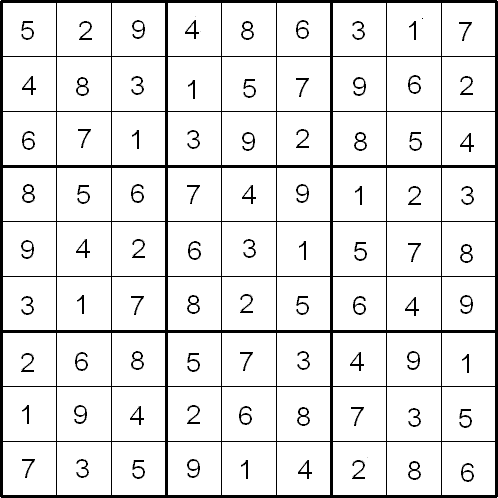#### You may also like### Not a Polite Question

When asked how old she was, the teacher replied: My age in years is not prime but odd and when reversed and added to my age you have a perfect square...### Whole Numbers Only

Can you work out how many of each kind of pencil this student bought?### Symmetricality

Add up all 5 equations given below. What do you notice? Solve the system and find the values of a, b, c , d and e. b + c + d + e = 4 a + c + d + e = 5 a + b + d + e = 1 a + b + c + e = 2 a + b + c + d = 0